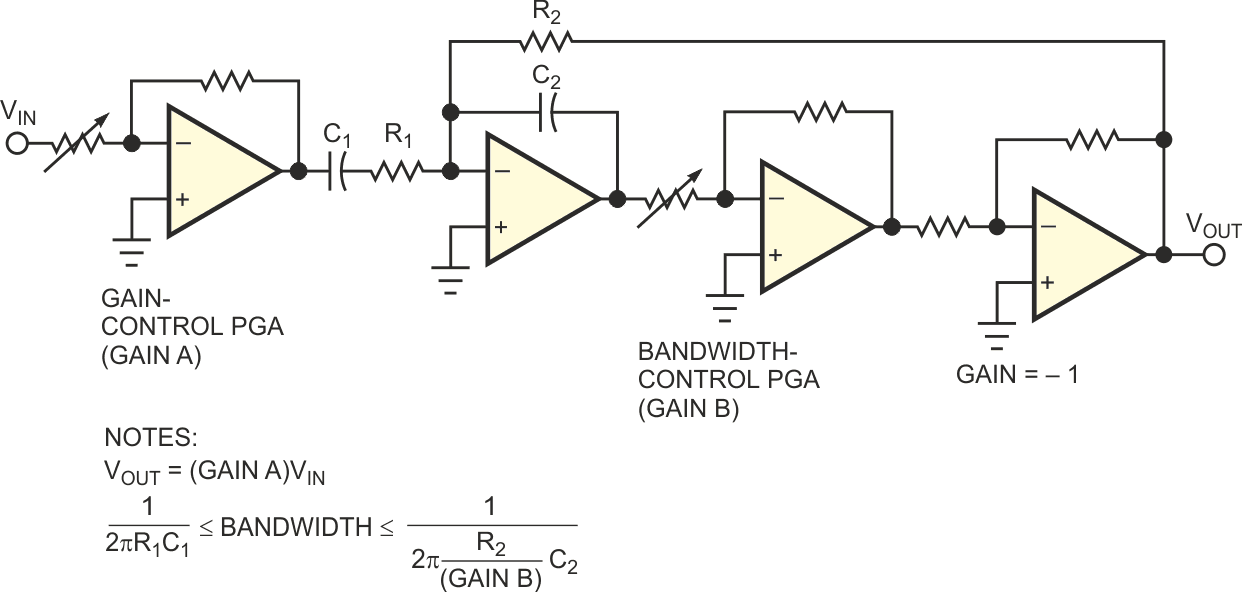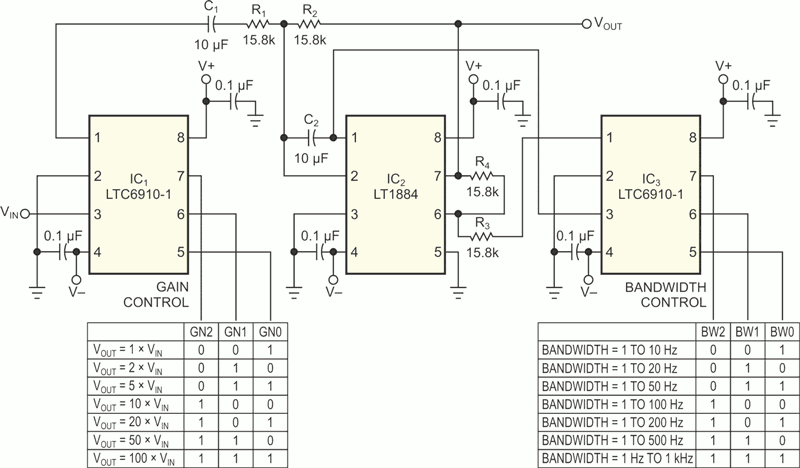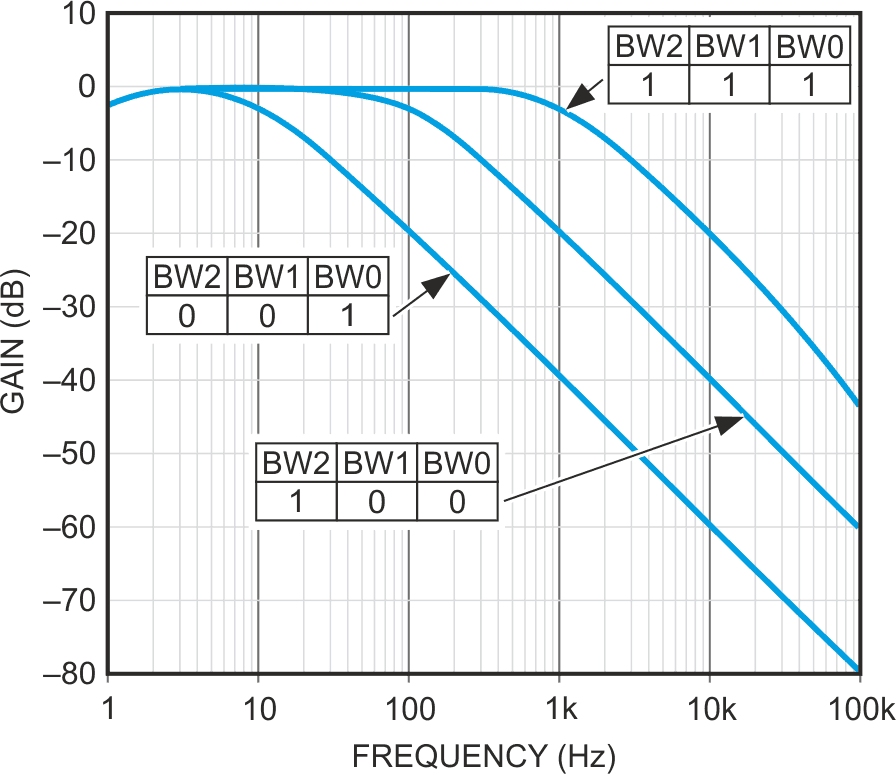# Low-noise ac amplifier has digital control of gain and bandwidth

## Analog Devices LT1884 LTC6910-1

Philip Karantzalis

EDN

In low-noise analog circuits, a high-gain amplifier serves at the input to increase the SNR. The input signal level determines the input-stage gain; low-level signals require the highest gain. It is also standard practice in low-noise analog-signal processing to make the circuit's bandwidth as narrow as possible to pass only the useful input-signal spectrum. The optimum combination of an amplifier's gain and bandwidth is the goal of a low-noise design. In a data-acquisition system, digital control of gain and bandwidth provides dynamic adjustment to variations in input-signal level and spectrum. Figure 1 shows a simplified circuit for an ac amplifier with control of both gain and bandwidth. The amplifier's input is a PGA (programmable-gain amplifier) providing gain control (Gain A). Following the input PGA is a first-order highpass filter formed with capacitor C1 and input resistor R1 of an integrator circuit. Inside the integrator's feedback path, the gain of a second PGA (Gain B) multiplies the integrator's –3-dB frequency, thus providing bandwidth control.Figure 1. This ac-amplifier configuration offers both gain and bandwidth control.

Figure 2 shows a complete circuit implementation using two LTC6910-1 digitally controlled PGAs and an LT1884 dual op amp. The input LTC6910-1, IC1, provides digital gain control from 1 to 100 using a 3-bit digital input to select gains of 1, 2, 5, 10, 20, 50, and 100. The circuit's lower –3-dB frequency is fixed and set to 1 Hz. A second LTC6910-1, IC3, is inside an LT1884-based (IC2) integrator loop. The integrator's digital gain control becomes digital bandwidth control, which provides an upper –3-dB frequency control of 10 Hz to 1 kHz.Figure 2. This detailed implementation of the circuit in Figure 1 operates with dual power supplies.

The circuit's low-noise LT1884 op amp and LTC6910-1 (9 nV/√Hz for each device) combine to provide high SNR. For example, the SNR is 76 dB for a 10-mV peak-to-peak signal with a gain of 100 and 100-Hz bandwidth or 64 dB for a 100-mV peak-to-peak signal with a gain of 10 and 1-kHz bandwidth. With an LT1884 dual op amp (gain-bandwidth product of 1 MHz), the circuit's upper frequency response can increase to 10 kHz by reducing the value of C2. (The lower –3-dB frequency increases by reducing the value of C1.) The circuit in Figure 2 operates with ±5.5 V dual power supplies. You can convert it to a single-supply 2.7 to 10 V circuit by grounding Pin 4 of IC1, IC2, and IC3; connecting a 1-µF capacitor from Pin 2 of IC1 to ground; and connecting Pin 2 of IC1 to pins 3 and 5 of IC2 and Pin 2 of IC3. Figure 3 shows the frequency response of the circuit in Figure 2 with unity gain and three digital bandwidth-control inputs.Figure 3. The frequency response of Figure 2's circuit shows unity gain and three digital bandwidth-control inputs.

## Materials on the topic

EDN